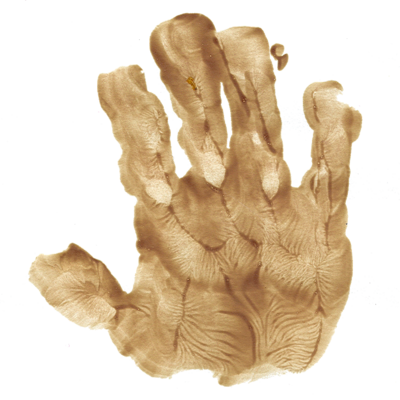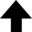A ray of light follows the path between two points that requires the least amount of time.Pierre de Fermat

Our everyday experience with light naturally leads to the concept of light rays. The propagation of light rays can be formulated in terms of a variational principle known as Fermat's principle of least time, which is the basis of classical or geometrical optics. As shown in the Notes, this principle states that the path taken between two points A and B by a light ray is the path that can be traversed in the least amount of time. Put another way: because the speed of light $v$ is constant (expressed as the ratio of the speed of light in vacuum $c$ to the index of refraction $n$) along any path within a given homogeneous medium, the path of shortest time is a straight line. This program uses Fermat's principle and a simple Monte Carlo method to find the path of a light ray.

Interestingly, the principle of least time—or, more broadly, least action—has inspired mathematicians to formulate new methods in number theory related to the rational solutions of Diophantine equations: Secret Link Uncovered Between Pure Math and Physics.

## The Law of Refraction

This program applies a simple variational Monte Carlo method to Fermat's principle of least time in geometrical optics.
 Index of refraction $n_1$ in the left half of the system: Vacuum Air Helium Hydrogen Carbon dioxide Water Ethanol Olive oil Ice Soda-lime glass PMMA (Plexiglas) Crown glass Flint glass Diamond Index of refraction $n_2$ in the right half of the system: Vacuum Air Helium Hydrogen Carbon dioxide Water Ethanol Olive oil Ice Soda-lime glass PMMA (Plexiglas®) Crown glass Flint glass Diamond
0%
Current data point: